# Qlik Design Blog

All about product and Qlik solutions: scripting, data modeling, visual design, extensions, best practices, etc.

Announcements
QlikWorld May 10-12, Online and Free! REGISTER NOWEmployee

## The Above Function

The Above() function is a very special function. It is neither an aggregation function, nor a scalar function. Together with some other functions, e.g. Top(), Bottom() and Below(), it forms a separate group of functions: Chart inter-record functions. These functions have only one purpose: To get values from other rows within the same chart.

The basic construction is the following:

Above( Sum( Sales ) )

This will calculate the sum of sales, but for the row above.

The most common use case is when you want to compare the value of a specific row with the value of the previous row; e.g. this month’s sales compared to last month’s sales.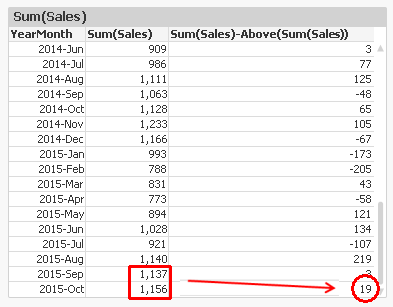Another use case is when you want to calculate rolling averages. Then you need to use the second and third parameter; the offset and the number of cells. Below, I use

Above( Sum( Sales ), 0, 12 )

The function will return 12 rows: the value for current row and the 11 rows above. This means that you need to wrap it in a range function in order to merge all values to one value. In this case, I use RangeAvg() to calculate the average of the 12 rows.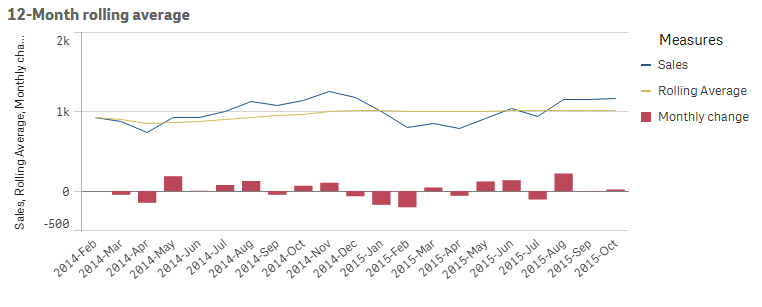However, both the above solutions have a flaw: They don’t take excluded values into account. For example, if April is excluded due to a selection, the previous month of May becomes March, which probably isn’t what you want.

To correct this, you need to make the chart show all months, also the excluded ones. In QlikView, you have a chart option “Show all values” that you can use. A method that works also in Qlik Sense, is to add zero to all values, also for the excluded dimensional values:

Sum( Sales ) + Sum( {1} 0 )

Make sure to “Show zero values”.

You can also use the Above() function inside an Aggr() function. Remember that the Aggr() produces a virtual table, and the Above() function can of course operate in this table instead. This opens tremendous new possibilities.

First, you can make the same calculations as above, by using

Only(Aggr(Above(Sum({1} Sales)), YearMonth))

Only(Aggr(RangeAvg(Above(Sum({1} Sales),0,12)), YearMonth))

Note the Set Analysis expression in the inner aggregation function. The {1} ensures that all values in the virtual table are calculated, so that the Above() function can fetch also the excluded ones. Using {1} is maybe too drastic – it is often better to use a Set expression that clears only some fields, e.g. {\$<YearMonth=>}.

Further, you can have a virtual table that is sorted differently from the chart where the expression is displayed. For example, the expression

Aggr(Above(Sum(Sales)),Year,Month)

displays the value from the previous month from the same year. But if you change the order of the dimensions, as in

Aggr(Above(Sum(Sales)),Month,Year)

the expression will display the value from the same month from the previous year. The only difference is the order of the dimensions. The latter expression is sorted first by Month, then by Year. The result can be seen below: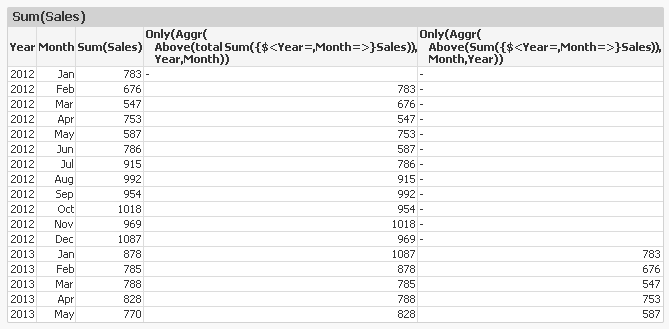An Aggr() table is always sorted by the load order of the dimensions, one by one. This means that you can change the meaning of Above() by changing the order of the dimensions.

With this, I hope that you understand the Above() function better.

HIC

Further reading related to this topic:

Accumulative Sums

Accumulations

Pitfalls of the Aggr functionMVP

Useful information as usual...

Like below expression

Sum( Sales ) + Sum( {1} 0 )

6,677 Views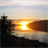Creator III

Thanks so much.. Really simple and hellpful

6,677 Views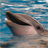Partner

Make a note of the date the tickets are available…

6,677 ViewsMVP

Great post Henric!

I think we should emphasize that the order of dimension values in an aggr() virtual table is load order, so

when we use something like

Aggr(Above(Sum(Sales)),Year,Month)

Month field values should have load order in chronological order, to give meaningful results when using chart inter record functions like above().

Maybe it's good to add this one also to the 'Pitfalls of the aggr function' blog, too, since I have seen many users struggle with this (though Henric discussed this in the comments section of that blog post).

Thanks again, Henric.

6,677 ViewsSpecialist

Excellent post (as usual)

Here is one of my YouTube videos based on another useful HIC post about Gantt Charts that shows another use for functions such as Above and RangeMax.

Getting Started with QlikView Gantt Charts - Rangemax function - YouTube

Thanks

Mark

http://techstuffy.tv

6,677 ViewsNot applicable

Wonderful way of explanation. Like it very much and appriciated.

Thanks and Happy New Year

6,677 ViewsNot applicable

Henric,

Pavel.

3,516 ViewsNot applicable

if ((Sum({<Article_No={'*'}-{''},Seasonwise={'Current'}>} net_qty ))<>0,

(
Sum({<Article_No={'*'}-{''},Seasonwise={'Current'}>} net_qty)),

aggr(Above( Sum({<Article_No={'*'}-{''},Seasonwise={'Current'}>} net_qty )),Entity,GLZone,Store_Code,Rolling_Month))

aggr function not working in this.  if i am trying 1 in else condition it is showing 1 , if i am trying only above statement it is working for first two months. any1 can help

3,516 ViewsEmployee

I suggest you enter your question in a separate thread. You will get many more answers that way.

• What do you want to achieve with "Article_No={'*'}-{''}"? Exclude NULLs? Then it's better to use "Article_No={"*"}".
• The Aggr() function must be wrapped in an aggregation function. It returns multiple values.

HIC

3,516 Views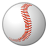Specialist

Recently I was looking something about Above function and thanks to Henric for share his knowledge I found a solution.  But I have a couple of comments over the solution of months with zero values.

First is that if you "show all values" it may appears dates that you may don't want to show.  And second I can see the change adding 0 (Sum{1} 0) to the result.

3,516 Views